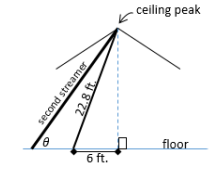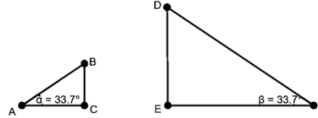# MA.912.T.1.1Export Print
Define trigonometric ratios for acute angles in right triangles.

### Clarifications

Clarification 1: Instruction includes using the Pythagorean Theorem and using similar triangles to demonstrate that trigonometric ratios stay the same for similar right triangles.

Clarification 2: Within the Geometry course, instruction includes using the coordinate plane to make connections to the unit circle.

Clarification 3: Within the Geometry course, trigonometric ratios are limited to sine, cosine and tangent.

General Information
Subject Area: Mathematics (B.E.S.T.)
Strand: Trigonometry
Status: State Board Approved

## Benchmark Instructional Guide

### Terms from the K-12 Glossary

• Angle
• Hypotenuse
• Right Triangle

### Vertical Alignment

Previous Benchmarks

Next Benchmarks

### Purpose and Instructional Strategies

In grade 8, students solved problems involving similar triangles. In Geometry, students will use similarity in right triangles to develop the definition of trigonometric ratios. In later courses, trigonometric ratios will be used to define trigonometric functions on the unit circle and the real line.
• Instruction includes students developing the definitions for sine, cosine and tangent using ratios of side lengths in similar triangles and the Pythagorean Theorem.
• For example, given a set of similar right triangles each with one side length missing, students can find the missing side lengths using the Pythagorean Theorem. Additionally, students can determine the constant of proportionality among the similar triangles. Students can then calculate the trigonometric ratios for each triangle and conclude that the corresponding ratios are the same for each triangle. Lead students to conclude the trigonometric ratios correspond to the angle measure and that each angle measure of an acute angle can be assigned to a sine, cosine, and tangent using an appropriate right triangle.
• Instruction includes discussing the relationships between the sine, cosine and tangent of the two acute angles of a triangle.
• For example, given a right triangle ABC, where angle C is the right angle, students can determine that sin A = cos B, cos A = sin B and tan A = $\frac{\text{1}}{\text{tan B}}$. Students should realize that these equations are true as long as angle A and angle B are complementary angles.
• Instruction includes the use of technology, including a calculator, to show how the sine, cosine and tangent of any acute angle (and more) can be found.
• Within the Geometry course, the expectation is to use angle measures given in degrees and not in radians. Additionally, it is not the expectation for students to master the trigonometric ratios of secant, cosecant and cotangent within this course.
• It is customary to use Greek letters to represent angle measures (e.g., θ, α, β, γ).
• Instruction includes the connection to the unit circle.
• For example, given a right triangle on the coordinate plane with vertices at (0,0), ($x$, 0) and ($x$, $y$), and the length of the hypotenuse equal to 1. Students can find the sine, cosine and tangent of the acute angle that the hypotenuse forms with the $x$-axis.

### Common Misconceptions or Errors

• Students may misidentify the sides of triangles.
• For example, students may identify the hypotenuse as being the adjacent leg or confuse the adjacent and opposite sides.

• Provide students with a set of similar right triangles and their side lengths.
• Part A. Identify corresponding angles and label them as ∠A, ∠B and ∠C, with ∠C denoting the right angle.
• Part B. Write the following ratios for each one of the right triangles with respect to ∠A
opposite leg : hypotenuse, adjacent leg: hypotenuse and
opposite leg: adjacent leg. What do you notice?
• Part C. How do your ratios created in Part B relate to sine, cosine and tangent?

### Instructional Items

Instructional Item 1
• Belle is hanging streamers for her brother’s surprise birthday party. She secures two streamers of different lengths at the peak of the ceiling. The center of the floor is directly underneath the ceiling peak. The distance along the floor from the center of the room to where the first streamer is attached is 6 feet. The second streamer is attached to the floor further from the center of the floor than the first streamer.• The distance between the streamers is $x$ feet and the length of the second streamer is $y$ feet. The angle formed between the second streamer and the floor is θ. Select all of the equations that are true to the nearest tenth based on the diagram.
1. sin θ = $\frac{\text{22.0}}{\text{y}}$
2. sin θ = $\frac{\text{22.8}}{\text{y}}$
3. tan θ = $\frac{\text{22.0}}{\text{6}}$
4. cos θ = $\frac{\text{x}}{\text{y}}$
5. cos θ = $\frac{\text{x+6}}{\text{22.8}}$
6. tan θ = $\frac{\text{22.0}}{\text{x+6}}$
7. sin θ = $\frac{\text{22.0}}{\text{22.8}}$
8. tan θ = $\frac{\text{22.8}}{\text{x}}$

Instructional Item 2
• Given the diagram below showing two right triangles, complete the following statements.• Statement A. sin 33.7° =
• Statement B. sin 33.7° = $\frac{\text{}}{\text{DF}}$
• Statement C. $\frac{\text{BC}}{\text{AC}}$ = $\frac{\text{}}{\text{}}$

*The strategies, tasks and items included in the B1G-M are examples and should not be considered comprehensive.

## Related Courses

This benchmark is part of these courses.
1200400: Foundational Skills in Mathematics 9-12 (Specifically in versions: 2014 - 2015, 2015 - 2022, 2022 and beyond (current))
1206310: Geometry (Specifically in versions: 2014 - 2015, 2015 - 2022, 2022 and beyond (current))
1206320: Geometry Honors (Specifically in versions: 2014 - 2015, 2015 - 2022, 2022 and beyond (current))
1206315: Geometry for Credit Recovery (Specifically in versions: 2014 - 2015, 2015 - 2022, 2022 and beyond (current))
7912065: Access Geometry (Specifically in versions: 2015 - 2022, 2022 and beyond (current))

## Related Access Points

Alternate version of this benchmark for students with significant cognitive disabilities.
MA.912.T.1.AP.1: Select a trigonometric ratio for acute angles in right triangles limited to sine or cosine.

## Related Resources

Vetted resources educators can use to teach the concepts and skills in this benchmark.

## Formative Assessments

The Sine of 57:

Students are asked to explain what a given sine ratio indicates about a right triangle and if the sine of a specific value varies depending on the right triangle.

Type: Formative Assessment

The Cosine Ratio:

Students are asked to compare the ratio of corresponding sides of two triangles and to explain how this ratio is related to the cosine of a given angle.

Type: Formative Assessment

Sine and Cosine:

Students are asked to explain the relationship between sine and cosine of the acute angles of a right triangle.

Type: Formative Assessment

Right Triangle Relationships:

Students are given the sine and cosine of angle measures and asked to identify the sine and cosine of their complements.

Type: Formative Assessment

Finding Sine:

Students are asked to explain the relationship between sine and cosine of complementary angles.

Type: Formative Assessment

Patterns in the 30-60-90 Table:

Students are asked to use 30-60-90 triangle relationships to observe and explain the relationship between sin 30 and cos 60 (or sin 60 and cos 30).

Type: Formative Assessment

## Lesson Plans

Discovering Trigonometric Ratios:

Students investigate and discover trigonometric ratios by drawing and measuring side lengths for five triangles that have equivalent angle measure. Students collect, analyze, and discuss data to draw conclusions. This is the introductory lesson to facilitate student discovery of trigonometric ratios and allows students to secure a solid foundation before the use of trigonometry to find missing sides. This lesson has students solving application problems by finding an unknown angle based on length measurements.

Type: Lesson Plan

Let's Get "Triggy":

This lesson helps students discover trigonometric ratios and how to apply them to find the measure of sides and angles of a right triangle.  Students will think about problems, discuss concepts with a partner and then share ideas with the class. Students will collaborate and offer supportive coaching to help deepen each other’s understanding.

Type: Lesson Plan

## MFAS Formative Assessments

Finding Sine:

Students are asked to explain the relationship between sine and cosine of complementary angles.

Patterns in the 30-60-90 Table:

Students are asked to use 30-60-90 triangle relationships to observe and explain the relationship between sin 30 and cos 60 (or sin 60 and cos 30).

Right Triangle Relationships:

Students are given the sine and cosine of angle measures and asked to identify the sine and cosine of their complements.

Sine and Cosine:

Students are asked to explain the relationship between sine and cosine of the acute angles of a right triangle.

The Cosine Ratio:

Students are asked to compare the ratio of corresponding sides of two triangles and to explain how this ratio is related to the cosine of a given angle.

The Sine of 57:

Students are asked to explain what a given sine ratio indicates about a right triangle and if the sine of a specific value varies depending on the right triangle.

## Student Resources

Vetted resources students can use to learn the concepts and skills in this benchmark.

## Parent Resources

Vetted resources caregivers can use to help students learn the concepts and skills in this benchmark.